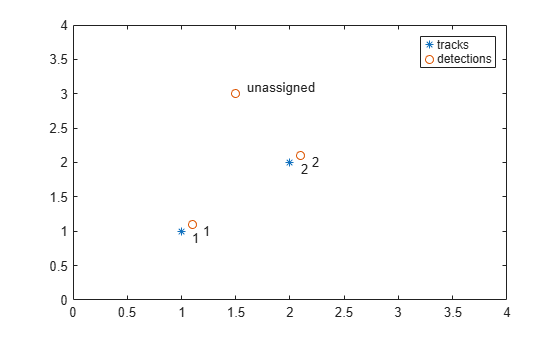# assignmunkres

Munkres global nearest neighbor assignment algorithm

## Syntax

``[assignments,unassignedrows,unassignedcolumns] = assignmunkres(costmatrix,costofnonassignment)``

## Description

example

````[assignments,unassignedrows,unassignedcolumns] = assignmunkres(costmatrix,costofnonassignment)` returns a table of `assignments` of detections to tracks using the Munkres algorithm. The Munkres algorithm obtains an optimal solution to the global nearest neighbor (GNN) assignment problem. An optimal solution minimizes the total cost of the assignments.The cost of each potential assignment is contained in the cost matrix, `costmatrix`. Each matrix entry represents the cost of a possible assignments. Matrix rows represent tracks and columns represent detections. All possible assignments are represented in the cost matrix. The lower the cost, the more likely the assignment is to be made. Each track can be assigned to at most one detection and each detection can be assigned to at most one track. If the number of rows is greater than the number of columns, some tracks are unassigned. If the number of columns is greater than the number of rows, some detections are unassigned. You can set an entry of `costmatrix` to `Inf` to prohibit an assignment.`costofnonassignment` represents the cost of leaving tracks or detections unassigned. Higher values increase the likelihood that every existing object is assigned.The function returns a list of unassigned tracks, `unassignedrows`, and a list of unassigned detections, `unassignedcolumns````

## Examples

collapse all

Use `assignMunkres` to assign three detections to two tracks.

`tracks = [1,1; 2,2];`

Assume three detections are received. At least one detection will not be assigned.

`dets = [1.1, 1.1; 2.1, 2.1; 1.5, 3];`

Construct a cost matrix by defining the cost of assigning a detection to a track as the Euclidean distance between them. Set the cost of non-assignment to 0.2.

```for i = size(tracks, 1):-1:1 delta = dets - tracks(i, :); costMatrix(i, :) = sqrt(sum(delta .^ 2, 2)); end costofnonassignment = 0.2;```

Use the Auction algorithm to assign detections to tracks.

```[assignments, unassignedTracks, unassignedDetections] = ... assignmunkres(costMatrix,costofnonassignment);```

Display the assignments.

`disp(assignments)`
``` 1 1 2 2 ```

Show that there are no unassigned tracks.

`disp(unassignedTracks)`

Display the unassigned detections.

`disp(unassignedDetections)`
``` 3 ```

Plot detection to track assignments.

```plot(tracks(:, 1), tracks(:, 2), '*', dets(:, 1), dets(:, 2), 'o') hold on xlim([0, 4]) ylim([0, 4]) legend('tracks', 'detections') assignStr = strsplit(num2str(1:size(assignments,1))); text(tracks(assignments(:, 1),1) + 0.1, ... tracks(assignments(:, 1),2) - 0.1, assignStr); text(dets(assignments(:, 2),1) + 0.1, ... dets(assignments(:, 2),2) - 0.1, assignStr); text(dets(unassignedDetections(:),1) + 0.1, ... dets(unassignedDetections(:),2) + 0.1, 'unassigned');```The track to detection assignments are:

1. Detection 1 is assigned to track 1.

2. Detection 2 is assigned to track 2.

3. Detection 3 is not assigned.

## Input Arguments

collapse all

Cost matrix, specified as an M-by-N matrix. M is the number of tracks to be assigned and N is the number of detections to be assigned. Each entry in the cost matrix contains the cost of a track and detection assignment. The matrix may contain `Inf` entries to indicate that an assignment is prohibited. The cost matrix cannot be a sparse matrix.

Data Types: `single` | `double`

Cost of non-assignment, specified as a scalar. The cost of non-assignment represents the cost of leaving tracks or detections unassigned. Higher values increase the likelihood that every object is assigned. The value cannot be set to `Inf`.

Data Types: `single` | `double`

## Output Arguments

collapse all

Assignment of detections to track, returned as an integer-valued L-by-2 matrix where L is the number of assignments. The first column of the matrix contains the assigned track indices and the second column contains the assigned detection indices.

Data Types: `uint32`

Indices of unassigned tracks, returned as an integer-valued P-by-1 column vector.

Data Types: `uint32`

Indices of unassigned detections, returned as an integer-valued Q-by-1 column vector.

Data Types: `uint32`

 Samuel S. Blackman and Popoli, R. Design and Analysis of Modern Tracking Systems. Artech House: Norwood, MA. 1999.